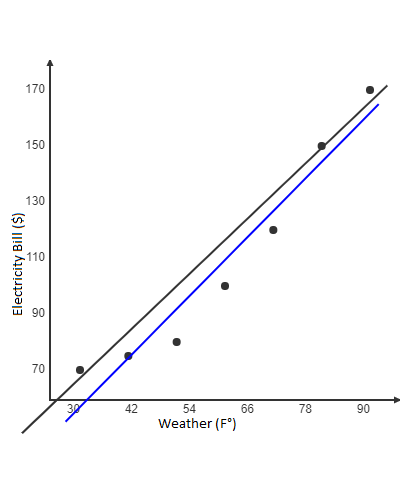Search

# Machine Learning Algorithm: Simple Linear Regression

Simple Linear Regression is a linear model that explains a linear relationship between the two quantitative variables, the input variable (dependent variable, on Y axis) and the output variable(independent variable, on X axis). When there is a single input variable (x), the method is referred to as Simple Linear Regression.Here is the equation for Linear Regression,

Y = a + bX

where,

Y is the dependent variable

X is the independent variable

b is the slop of the line

a is the Y-intercept

Lets get a better understanding on Linear Regression with an example:

Here is the predication of Electricity Bill per month based on the Weather.

Below is the data in which we have taken the electricity bill(Dependent variable, Y) per month and the corresponding average weather(Independent variable, X) of that particular month to find the Linear Regression equation/Line of Best fit.Once we get the model and Linear Regression equation we can predict the future electricity bill for a month based on that month's average weather (F°)Based on the data, we have got the Simple Linear Regression equation to be:

y = 1.75x + 4.285714

Intercept (a) = 4.285714

Slope (b) = 1.75.

Happy Learning :)

See All